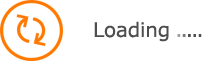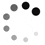Featured

# 16 oz Swiggy Stainless Steel Bottle

Item# 736241
 Choose
Back• Quantity: X
• Zip Code:
• Shipping:
•  Total:
•Back
You may also like:
Description
Item specs
Customization
Reviews
Description

• Screw On, Spill-Resistant Lid.
• Wide Mouth Opening.
• BPA-Free.
• Double Wall Construction For Insulation Of Hot And Cold Liquids.
• Vacuum Sealed.
• Keeps Drinks Cold Up To 24 Hours And Hot Up To 12 Hours.
• Meets FDA Requirements.
• Hand Wash Recommended.

Special Instructions:
Must be ordered in full carton quantities.

Item SpecsCustomizationReviewsViolette W.December 05, 2018
Product is great and the customer service is excellent!!
We recently purchased these for a company event and to hand out to new hires. the quality is good. we tested it for hot and cold beverages and it holds true to it's description. We ordered a sample prior to ordering and since there was a delay on receiving it from the shipping company (holidays); AnyPromo stepped up and provided 2-day shipping so our order would arrive as promised originally. They email and touch base regularly. Very thorough!
Did you find this review helpful?Nicolina M.November 28, 2018
It was perfect! Thanks!
It was perfect! Thanks! :)))))))))))))))))))))))))))))))))))))))))))))))))))))))))))))))))))))))))))))))))))))))))))))))))))))))))))))))))))))))))))))))))))))))))))))))))))))))))))))))))))))))))))))))
Did you find this review helpful?